Question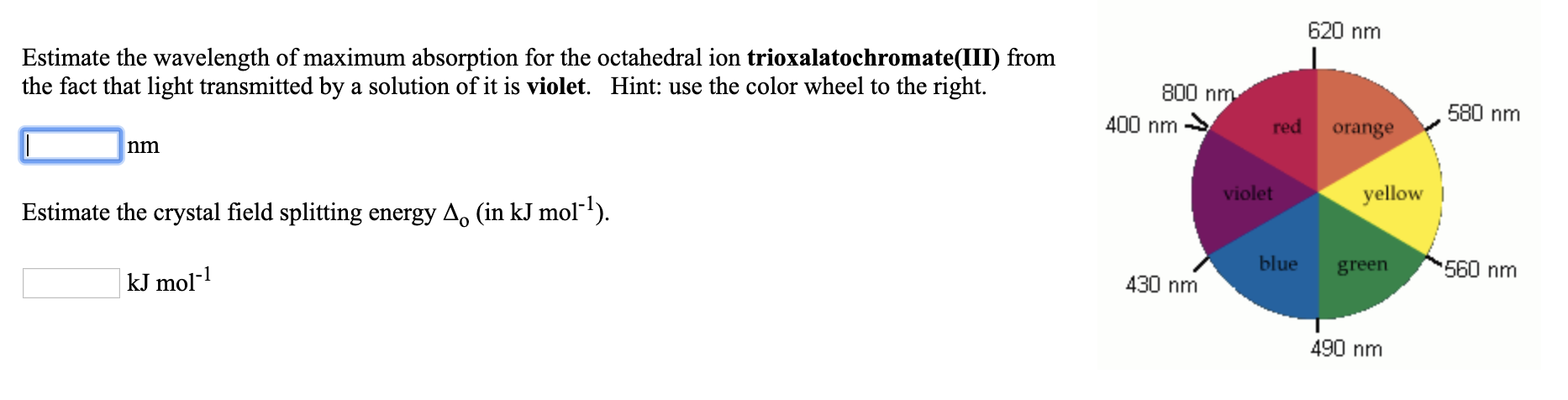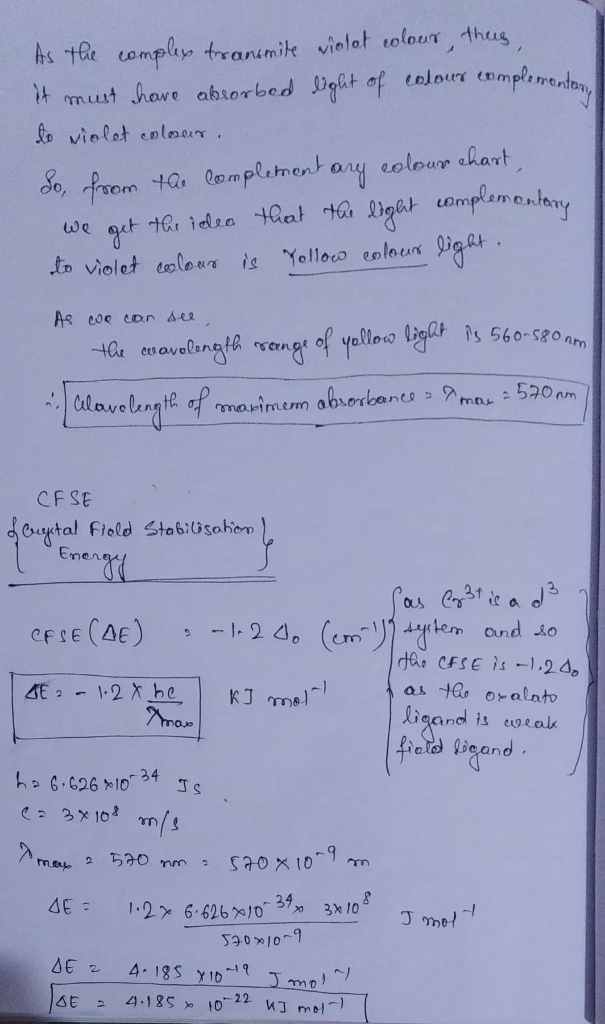#### Earn Coins

Coins can be redeemed for fabulous gifts.

Similar Homework Help Questions
• ### Estimate the wavelength of maximum absorption for the octahedral ion hexaamminecobalt(lII) from right the fact that...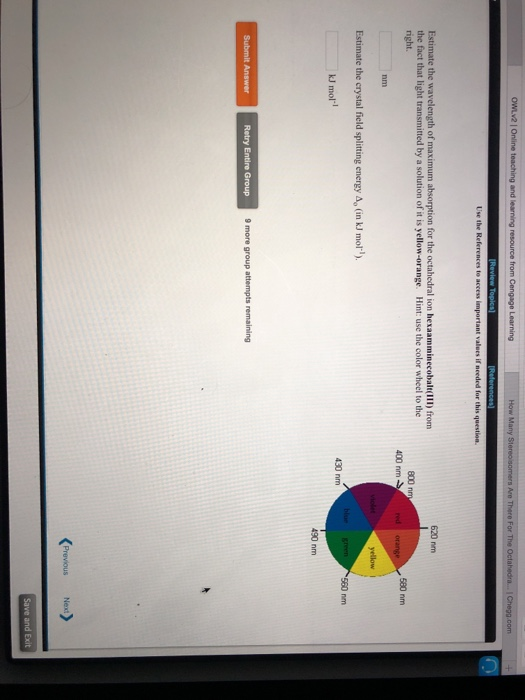Estimate the wavelength of maximum absorption for the octahedral ion hexaamminecobalt(lII) from right the fact that light transmitted by a solution of it is yellow-orange. Hint: use the color wheel to the 620 nm 800 nm 400 nm 580 nm nm Estimate the crystal field splitting energy Ap (in kJ mol) kJ mol-l 430 nm 490 nm Retry Entire Group 9 more group attempts remaining

• ### References Use the References to access important values if needed for this question. 620 nm Estimate...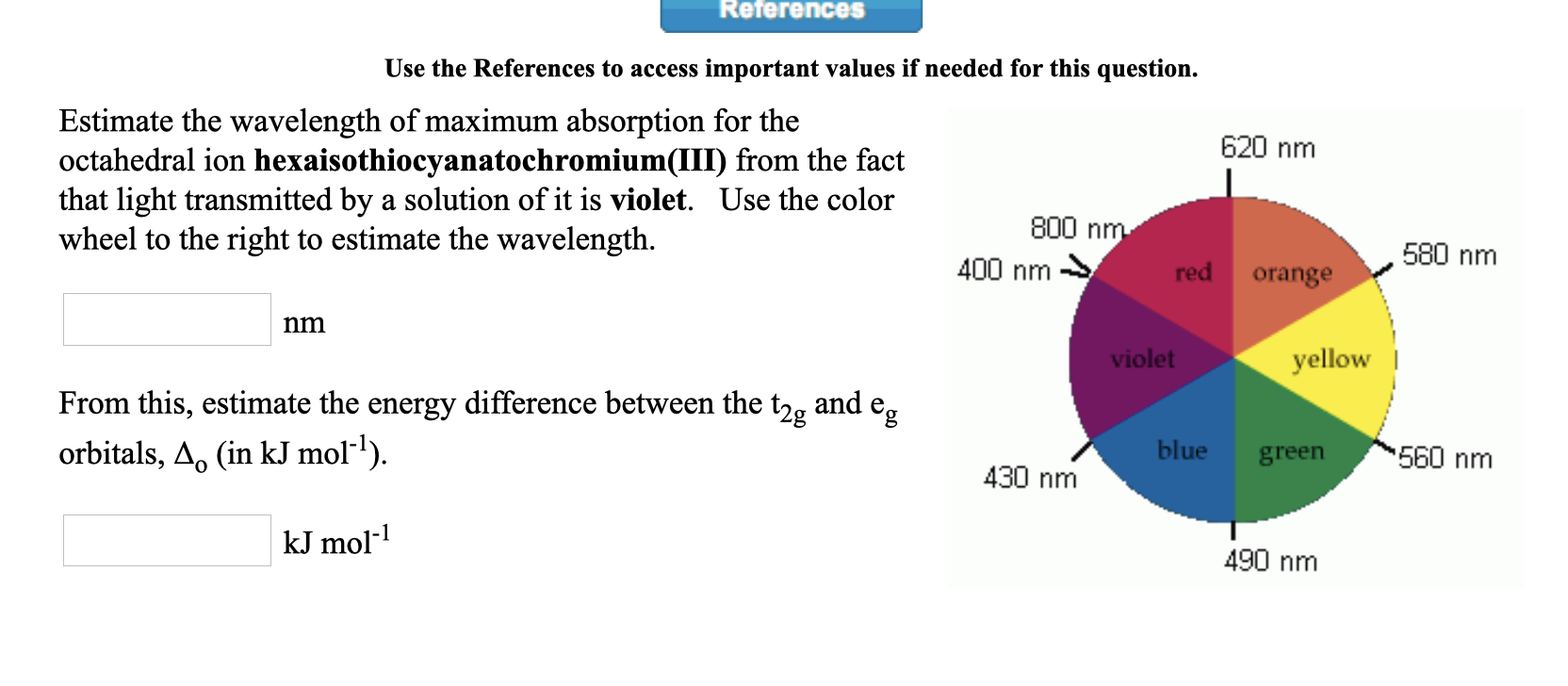References Use the References to access important values if needed for this question. 620 nm Estimate the wavelength of maximum absorption for the octahedral ion hexaisothiocyanatochromium(III) from the fact that light transmitted by a solution of it is violet. Use the color wheel to the right to estimate the wavelength. 800 nm 400 nm red orange 580 nm nm violet yellow From this, estimate the energy difference between the t2, and e, orbitals, A. (in kJ mol-?). blue green 560...

• ### Use the References to access important values if needed for this question. Estimate the wavelength of...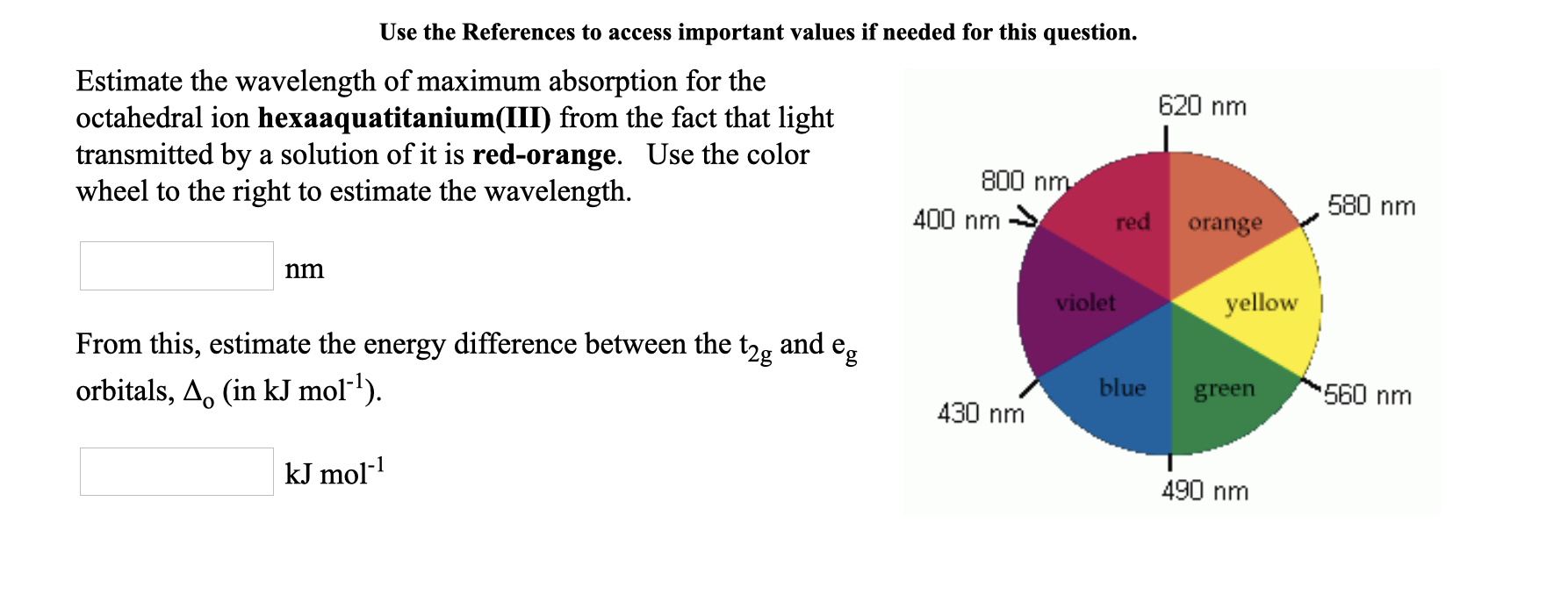Use the References to access important values if needed for this question. Estimate the wavelength of maximum absorption for the 620 nm octahedral ion hexaaquatitanium(III) from the fact that light transmitted by a solution of it is red-orange. Use the color 800 nm wheel to the right to estimate the wavelength. 400 nm → red orange ,580 nm nm violet yellow From this, estimate the energy difference between the t2g and en orbitals, A. (in kJ mol-?). blue green 560...

• ### An octahedral metal complex absorbs light with wavelength 580 nm. a. What is the crystal field...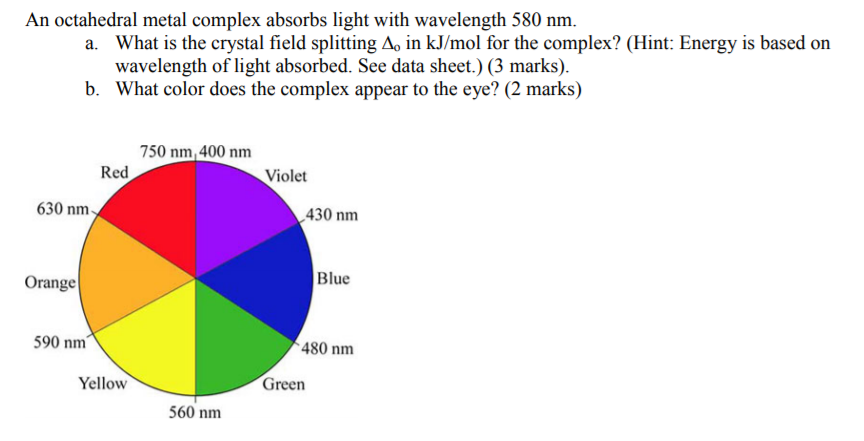An octahedral metal complex absorbs light with wavelength 580 nm. a. What is the crystal field splitting A, in kJ/mol for the complex? (Hint: Energy is based on wavelength of light absorbed. See data sheet.) (3 marks) b. What color does the complex appear to the eye? (2 marks) 750 nm, 400 nm Red Violet 630 nm 430 nm Blue Orange 590 nm 480 nm Yellow Green 560 nm

• ### Estimate the crystal field stabilization energy for the octahedral ion hexacyanocobaltate(III), if the wavelength of maximum...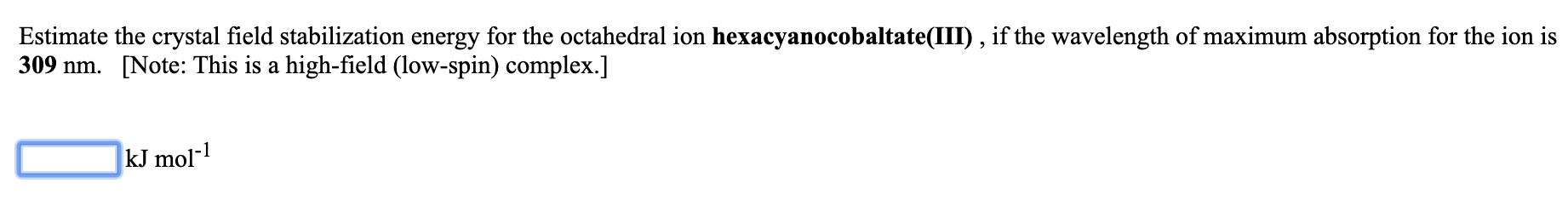Estimate the crystal field stabilization energy for the octahedral ion hexacyanocobaltate(III), if the wavelength of maximum absorption for the ion is 309 nm. [Note: This is a high-field (low-spin) complex.] kJ mol-1

• ### Estimate the crystal field stabilization energy for the octahedral ion hexafluorocobaltate(III), if the wavelength of maximum...

Estimate the crystal field stabilization energy for the octahedral ion hexafluorocobaltate(III), if the wavelength of maximum absorption for the ion is 710 nm.   [Note: This is a weak-field (high-spin) complex.] _____ kJ mol-1

• ### Estimate the crystal field stabilization energy for the octahedral ion hexacyanomanganate(III), if the wavelength of maximum...

Estimate the crystal field stabilization energy for the octahedral ion hexacyanomanganate(III), if the wavelength of maximum absorption for the ion is 600 nm. [Note: This is a high-field (low-spin) complex.] kJ mol-1

• ### 1) Estimate the crystal field stabilization energy for the octahedral ion hexaisothiocyanatotitanate(III), if the wavelength of...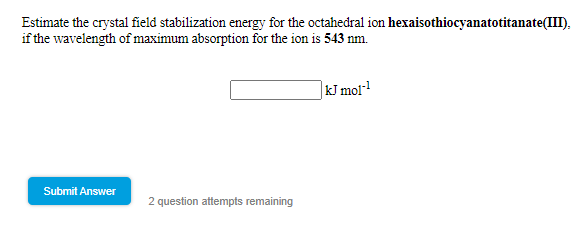1) Estimate the crystal field stabilization energy for the octahedral ion hexaisothiocyanatotitanate(III), if the wavelength of maximum absorption for the ion is 543 nm Estimate the crystal field stabilization energy for the octahedral ion hexaisothiocyanatotitanate(III). if the wavelength of maximum absorption for the ion is 543 nm. kJ moi? Submit Answer 2 question attempts remaining

• ### The electronic absorption spectrum UI (Cr(NH3)6]3+ (aq) is shown in Figure 11.5. One of the...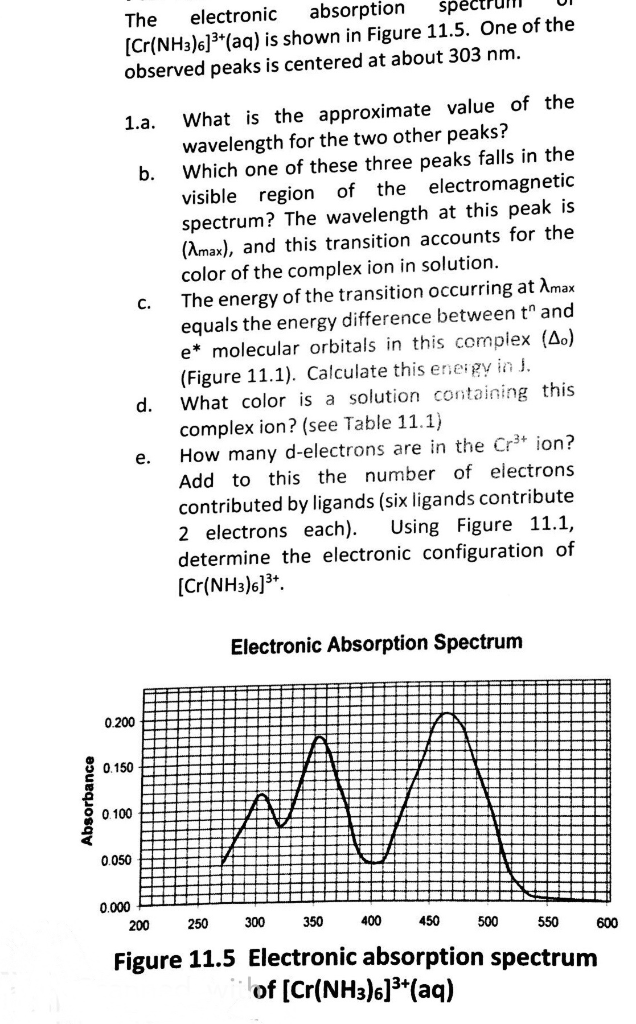The electronic absorption spectrum UI (Cr(NH3)6]3+ (aq) is shown in Figure 11.5. One of the observed peaks is centered at about 303 nm. 1.a. What is the approximate value of the wavelength for the two other peaks? Which one of these three peaks falls in the visible region of the electromagnetic spectrum? The wavelength at this peak is (Amax), and this transition accounts for the color of the complex ion in solution. The energy of the transition occurring at...

• ### Can you do Q6? EXERCISES 153 Woodward's rules, predict the absorption maximum for the 2-5 Using...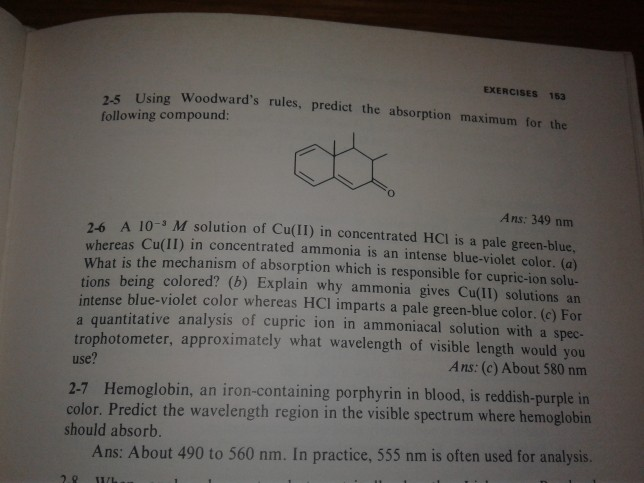Can you do Q6? EXERCISES 153 Woodward's rules, predict the absorption maximum for the 2-5 Using following compound: Ans: 349 nm 2-6 A 10- M solution of Cu(lI) in concentrated HCl is a pale green-blue. whereas Cu(II) in concentrated ammonia is an intense blue-violet color. (a) What is the mechanism of absorption which is responsible for cupric-ion solu- tions being colored? (b) Explain why ammonia gives Cu(II) solutions an intense blue-violet color whereas HCI imparts a pale green-blue color. (e)...